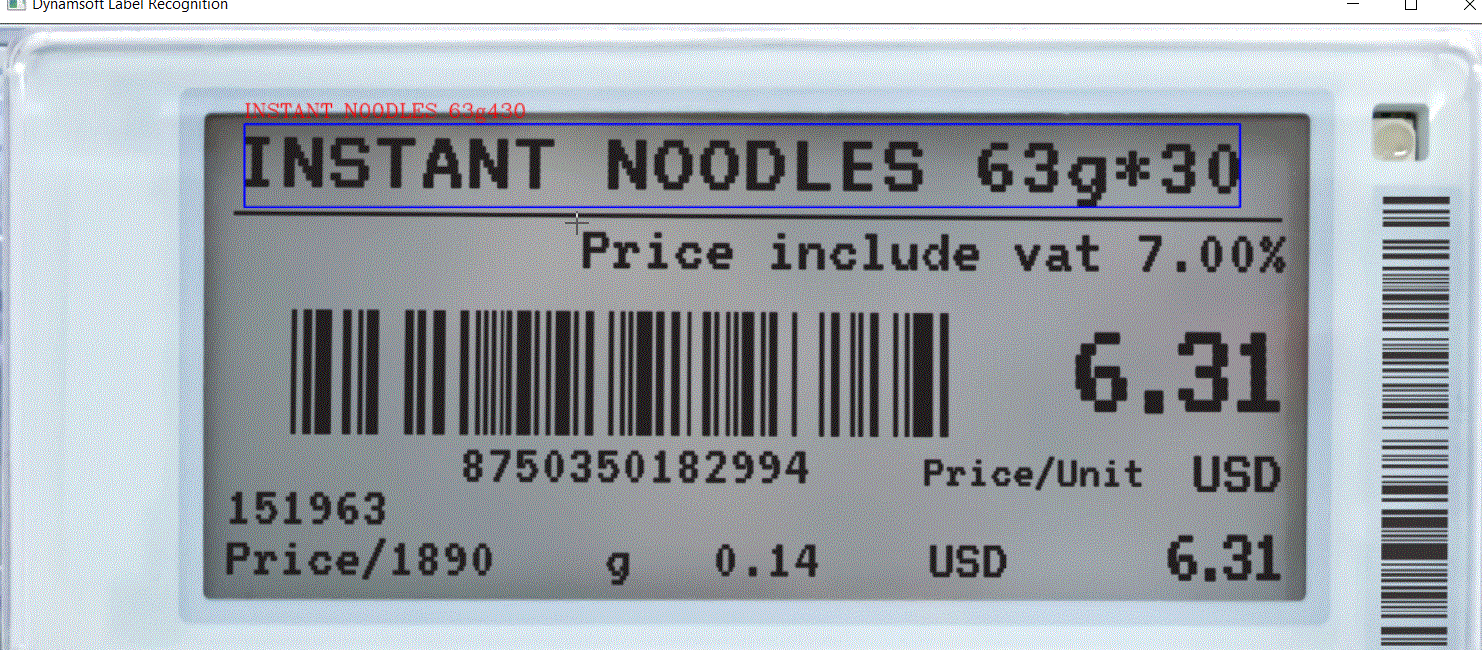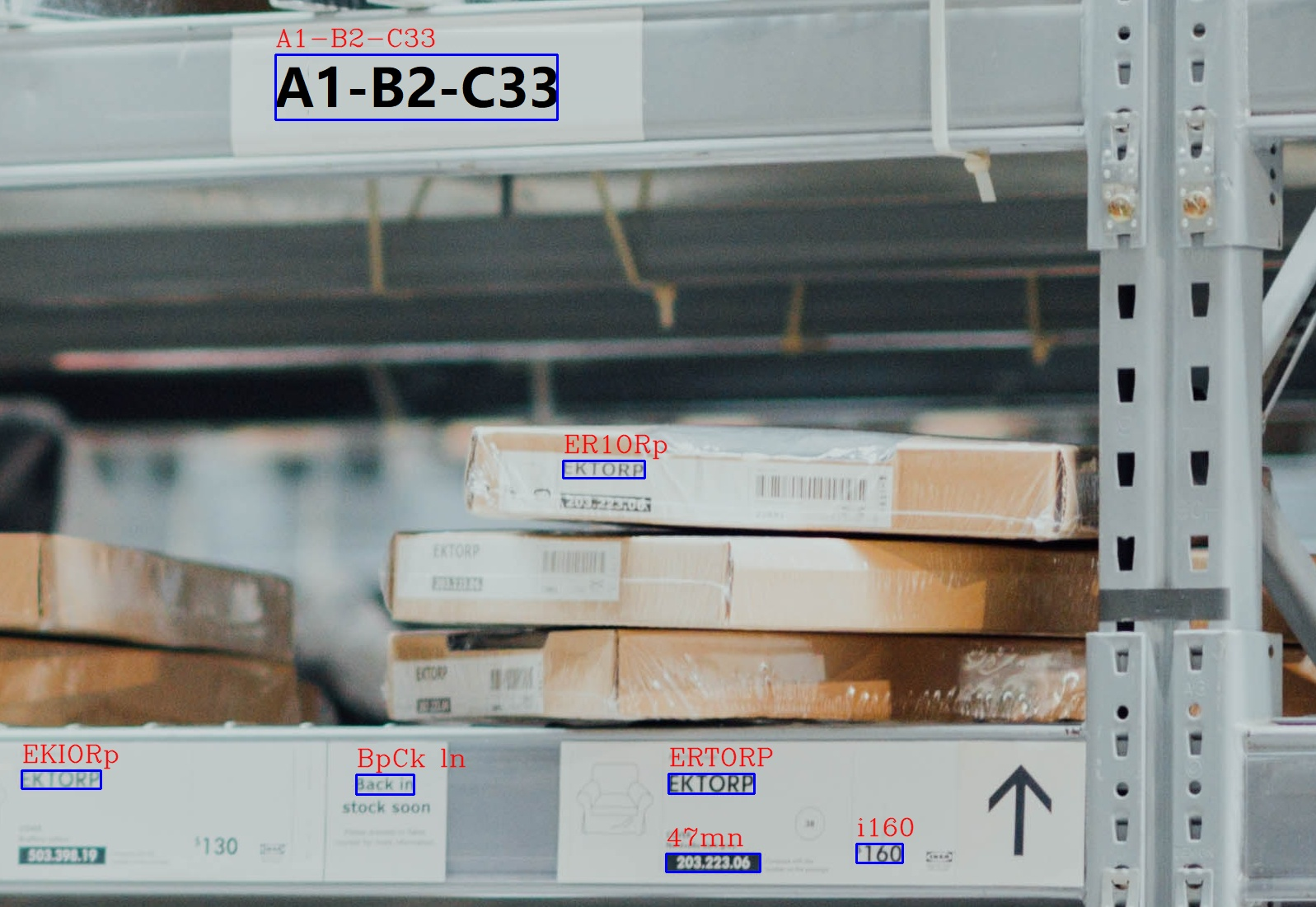×

# Text Recognition with Dynamsoft's OCR SDK

Dynamsoft Label Recognition (DLR) is an OCR SDK implemented in C/C++. The 1.0 Beta version was recently rolled out for `Windows` and `Linux` platforms. This article will demonstrate how to utilize the APIs of Dynamsoft Label Recognition to create a text recognition app on Windows.## Requirements

Get a free trial license and save it to `license.txt` file.

## Text Recognition in C/C++

Let’s get started with the command-line example (`DLR-C_CPP-1.0-Beta\DynamsoftLabelRecognition\Samples\LabelRecognitionDemo`) located in the DLR package.

There are 4 essential steps for extracting text from pictures:

1. Initialize the DLR object and set the trial license:

`````` CLabelRecognition dlr;
``````

`````` bool GetImagePath(char* pImagePath)
{
char pszBuffer = { 0 };
bool bExit = false;
size_t iLen = 0;
FILE* fp = NULL;
while (1)
{
printf("\r\n>> Step 1: Input your image file's full path:\r\n");
#if defined(_WIN32) || defined(_WIN64)
gets_s(pszBuffer, 512);
#else
fgets(pszBuffer, 512, stdin);
strtok(pszBuffer, "\n");
#endif
iLen = strlen(pszBuffer);
if (iLen > 0)
{
if (strlen(pszBuffer) == 1 && (pszBuffer == 'q' || pszBuffer == 'Q'))
{
bExit = true;
break;
}

memset(pImagePath, 0, 512);
if ((pszBuffer == '\"' && pszBuffer[iLen - 1] == '\"') || (pszBuffer == '\'' && pszBuffer[iLen - 1] == '\''))
memcpy(pImagePath, &pszBuffer, iLen - 2);
else
memcpy(pImagePath, pszBuffer, iLen);

#if defined(_WIN32) || defined(_WIN64)
int err = fopen_s(&fp, pImagePath, "rb");
if (err == 0)
{
fclose(fp);
break;
}
#else
fp = fopen(pImagePath, "rb");
if (fp != NULL)
{
break;
}
#endif
}
}
return bExit;
}
``````
3. Call the text recognition API:

`````` errorCode = dlr.RecognizeByFile(pszImageFile, "");
``````
4. Output OCR text results and corresponding corner points:

`````` DLRResultArray* pDLRResults = NULL;
dlr.GetAllDLRResults(&pDLRResults);
if (pDLRResults != NULL)
{
int rCount = pDLRResults->resultsCount;
printf("\r\nRecognized %d results\r\n", rCount);
for (int ri = 0; ri < rCount; ++ri)
{
printf("\r\Result %d :\r\n", ri);
DLRResult* result = pDLRResults->results[ri];
int lCount = result->lineResultsCount;
for (int li = 0; li < lCount; ++li)
{
printf("Line result %d: %s\r\n", li, result->lineResults[li]->text);
DLRPoint *points = result->lineResults[li]->location.points;
printf("x1: %d, y1: %d, x2: %d, y2: %d, x3: %d, y3: %d, x4: %d, y4: %d\r\n", points.x,
points.y, points.x, points.y, points.x, points.y, points.x, points.y);
}
}
}
else
{
printf("\r\nNo data detected.\r\n");
}
dlr.FreeDLRResults(&pDLRResults);
``````

By running the command-line app, you may have two questions:

• How to check whether the corner values are correct?
• How to set the recognition region properly in manual mode?

To tackle the two problems and get better user experiences, we can implement GUI with OpenCV based on the command-line sample.

## Drawing Bounding Boxes and Tracking Mouse Movement with OpenCV

To verify corner values, we can draw the corresponding rectangles on the image.

We use OpenCV APIs to read and show images:

``````const char* windowName = "Dynamsoft Label Recognition";
current = ori.clone();
namedWindow(windowName);
``````

Since the input data type has been changed to `Mat`, we use `RecognizeByBuffer()` instead of `RecognizeByFile()`:

``````DLRImageData data = {ori.step.p * imgHeight, ori.data, imgWidth, imgHeight, ori.step.p, DLR_IPF_RGB_888};
errorCode = dlr.RecognizeByBuffer(&data, "");
``````

As we get the OCR results, we can draw lines and text by corner values:

``````int rCount = pDLRResults->resultsCount;
printf("\r\nRecognized %d results\r\n", rCount);
for (int ri = 0; ri < rCount; ++ri)
{
printf("\rResult %d :\r\n", ri);
DLRResult* result = pDLRResults->results[ri];
int lCount = result->lineResultsCount;

for (int li = 0; li < lCount; ++li)
{
DLRPoint *points = result->lineResults[li]->location.points;
int x1 = points.x, y1 = points.y;
int x2 = points.x, y2 = points.y;
int x3 = points.x, y3 = points.y;
int x4 = points.x, y4 = points.y;
line( ori, Point(x1, y1), Point(x2, y2), cv::Scalar(255, 0, 0), thickness);
line( ori, Point(x2, y2), Point(x3, y3), cv::Scalar(255, 0, 0), thickness);
line( ori, Point(x3, y3), Point(x4, y4), cv::Scalar(255, 0, 0), thickness);
line( ori, Point(x4, y4), Point(x1, y1), cv::Scalar(255, 0, 0), thickness);
putText(ori, result->lineResults[li]->text, Point(x1, y1 - 10), FONT_HERSHEY_COMPLEX, 1, Scalar(0, 0, 255), 1, LINE_AA);
}
}
``````

We use `setMouseCallback()` to track mouse events:

``````void onMouse(int event, int x, int y, int f, void* ){
switch(event) {
case EVENT_LBUTTONDOWN:
clicked = true;
startPoint.x = x, startPoint.y = y;
break;
case EVENT_LBUTTONUP:
clicked = false;
doRegionDetection();
break;
case EVENT_MOUSEMOVE:
endPoint.x = x, endPoint.y = y;
break;
}

if (clicked)
{
drawRegion();
}
}

setMouseCallback(windowName, onMouse, NULL);
``````

When the mouse button is released and a region is selected, text recognition will be triggered:

``````void doRegionDetection()
{
char szErrorMsg;
double scale = 1.0;
if (hScale >= wScale && hScale > 1)
{
scale = hScale;
}
else if (hScale <= wScale && wScale > 1)
{
scale = wScale;
}
tagDLRPoint region = { {startPoint.x * scale, startPoint.y * scale},{endPoint.x * scale, startPoint.y * scale},{endPoint.x * scale, endPoint.y * scale},{startPoint.x * scale, endPoint.y * scale} };
if (SetDetectRegion(dlr, region, szErrorMsg, 512))
{
printf("\r\nSetDetectRegion Error: %s\r\n", szErrorMsg);
}

doOCR();
}
``````

Build and run the app on Windows :

``````mkdir build
cd build
cmake -DCMAKE_GENERATOR_PLATFORM=x64 -DENABLE_OPENCV=TRUE ..
cmake --build . --config release